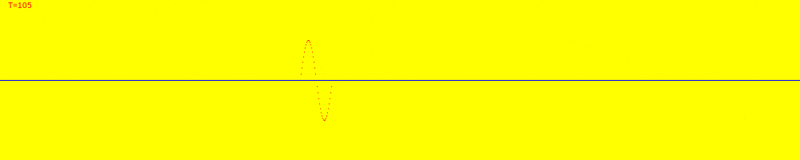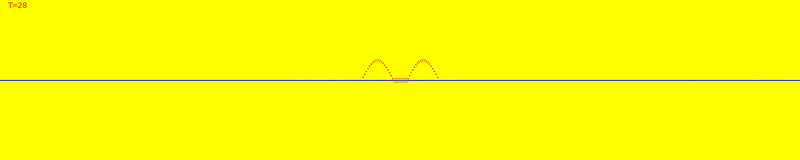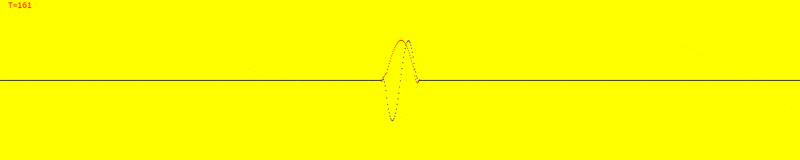# Hamiltonian V and T of a lattice?

• A
• James1238765
QuantumFieldTheory/chap6.pdfhttps://www.math.niu.edu/~james/QuantumFieldTheory/chap6.pdfThose should give you a good starting point.f
@PeterDonis another equivalent formulations is the operator Heisenberg picture, right? If it's equivalent, and we are discretising (ie removing all the theory and leaving pure numbers and artihmetic), why does the picture used matter?

another equivalent formulations is the operator Heisenberg picture, right?
If you are referring to the equivalence in non-relativistic QM between the Heisenberg and Schrodinger pictures, no, that does not exist in QFT either.

@James1238765 btw, the slides that @Frabjous referenced in post #6 also contain the answer to your question about time evolution (in the slide right before the one you posted a picture of in your OP).

discretising (ie removing all the theory and leaving pure numbers and artihmetic)
That's not a good description of what one is doing when discretizing a continuum theory.

•vanhees71
@PeterDonis the model is a toy model. I do not see how 100 years of QFT is needed to explain a toy model.
It's obvious, why one needs to explain a toy model when looking at your struggeling with it: It's to teach the next generation of physicists about the methodology how to tackle real-world QFT, and indeed QCD is a pretty delicate subject. So it's good to first study simple toy models first. As already Platon knew, there's no king's way to the wisdom. You have to go the whole way from the beginning to the end. There's no shortcut, and indeed you should learn physics from good text books and then from physics papers rather than from youtube videos!

•malawi_glenn
##\Phi## seems to be the fundamental object that exists on the lattice grid. H can be either defined or not defined, being an added constraint, but the time evolution is always defined in the end in terms of how ##\Phi## interacts between adjacent cells or adjacent times. Any method of defining the time evolution locally (in terms of adjacent cells or adjacent times) will invariably result in a wave-like structure and propagation of the field grid ##\Phi##.

1. $$\Phi_x^{t+1} = \Phi_x^t$$is a frozen field with no time evolution.

2. $$\Phi_x^{t+1} = \Phi_{x+1}^t$$is a field where everything moves in the same direction at a constant velocity c.

3. $$\Phi_x^{t+1} = \Phi_{x+1}^t + \Phi_{x-1}^t - \Phi_x^{t-1}$$is a classical wave equation field with 1 wave component ##\frac{d^2\Phi}{dt^2}=\frac{d^2\Phi}{dx^2}## where all waves disperse equally in all directions.

4. $$Re(\Phi_x^{t+1}) = - Im(\Phi_{x+1}^t - 2*\Phi_x^t + \Phi_{x-1}^t) + Re(\Phi_x^t)$$
$$Im(\Phi_x^{t+1}) = Re(\Phi_{x+1}^t - 2*\Phi_x^t + \Phi_{x-1}^t) + Im(\Phi_x^t)$$is the Schrodinger wave ##i\frac{d\Phi}{dt}=-\frac{d^2\Phi}{dx^2}## with 2 components Re and Im. To get localized wave packets that move in a specific direction, a minimum of 2 wave components are needed.

5. Boson 4-component waves and fermion 8-component waves may possess other properties not achievable using fewer components.

IIRC this dude has lots of information and test code on his website regarding lattice QFTs
https://latticeguy.net/

•Paul Colby, James1238765, vanhees71 and 1 other person
the time evolution is always defined in the end in terms of how ##\Phi## interacts between adjacent cells or adjacent times.
This is true, and the answer to the question I asked you in post #24 will help to clarify this. (Hint: "adjacent times" are "adjacent cells", in the time direction on the lattice.)

I can't tell how anything else in your post #46 relates to the lattice model you are asking about.

•malawi_glenn and vanhees71
I think now is a good time to close this thread. There is a lot of good information for the OP to consider and should take some time to review and reconsider his understanding of the subject.

Without much ado, I thank the OP for posing an interesting question and for everyone who has contributed here and now close the thread.

Jedi

•vanhees71 and berkeman